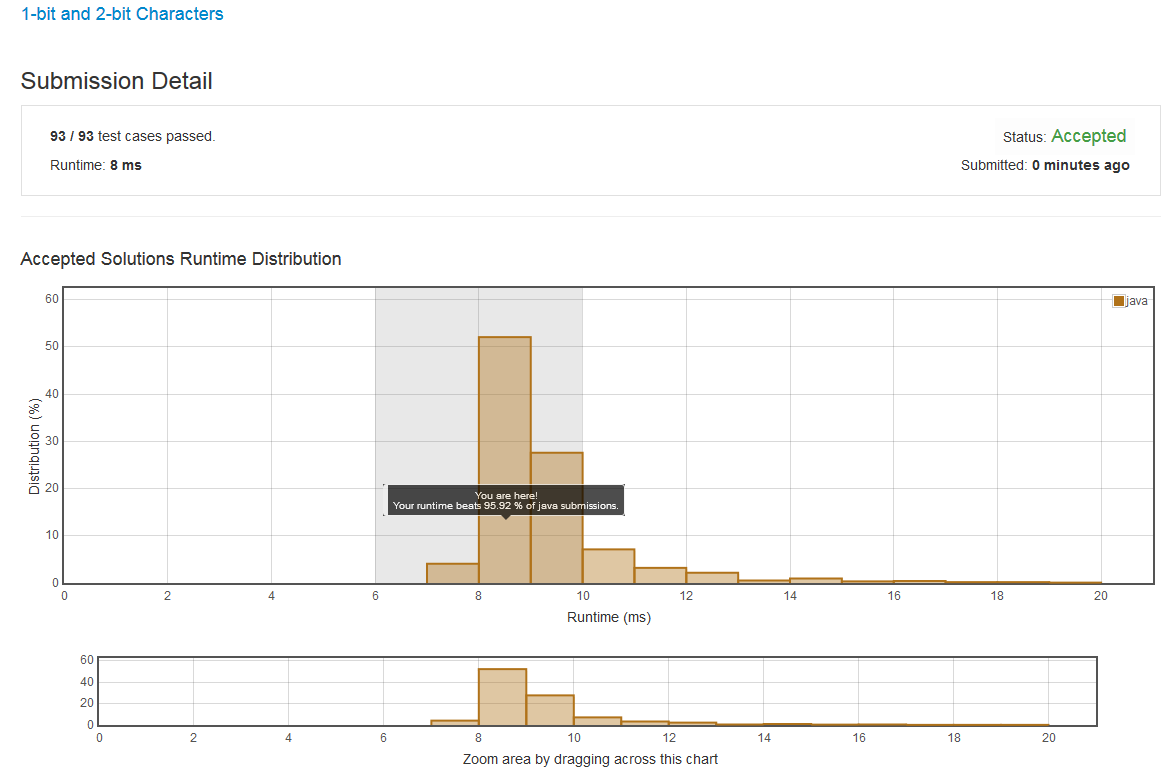# LeetCode 717. 1-bit and 2-bit Characters

We have two special characters. The first character can be represented by one bit 0. The second character can be represented by two bits (10 or 11).

Now given a string represented by several bits. Return whether the last character must be a one-bit character or not. The given string will always end with a zero.

Example 1:

Input:
bits = [1, 0, 0]
Output: True
Explanation:
The only way to decode it is two-bit character and one-bit character. So the last character is one-bit character.



Example 2:

Input:
bits = [1, 1, 1, 0]
Output: False
Explanation:
The only way to decode it is two-bit character and two-bit character. So the last character is NOT one-bit character.




Note:

• 1 <= len(bits) <= 1000.
• bits[i] is always 0 or 1.

class Solution {
public boolean isOneBitCharacter(int[] bits) {
int count = 0;
for(int i= bits.length-2; 0<=i; i--) {
if(bits[i] == 0) {
break;
} else {
count++;
}
}

if(count%2 == 0) {
return true;
} else {
return false;
}
}
}posted @ 2018-04-18 10:52  Zhao_Gang  阅读(93)  评论(0编辑  收藏  举报